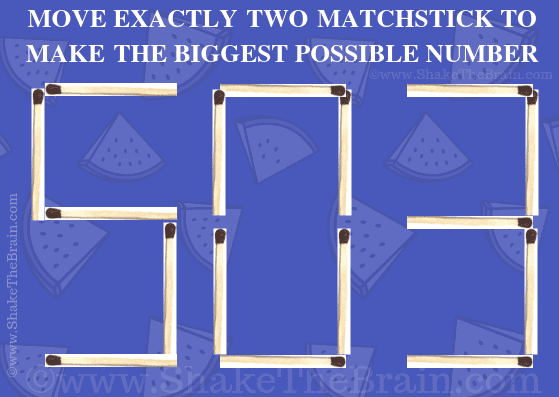## Friday, June 28, 2019

Here is a very interesting Matchstick Math Picture puzzle. Here one number formed using matchsticks. Your matchstick challenge is to move two matchsticks and then form the biggest number possible. Please do write your answer in the comment section.Can you solve this matchstick puzzle?

The answer to "Matchstick Math Picture Puzzle", can be viewed by clicking on the button. Please do give your best try before looking at the answer.

1.We can move the top and bottom of 0 to make the matchsticks look like : G 1 1 9

It's the 119th Graham number, https://en.wikipedia.org/wiki/Graham%27s_number

HUGE...

1.This is what we call out of box thinking :).

2.Exactly move two match stick The biggest number is 987

3.I think the answer is 987
Am I right

1.The number can be much bigger if one thinks outside the box to solve this #puzzle :)

2.4.I would move 2 match sticks from the end to before the 5 to make a 1 and therefore make 1507

5.6.7.8.9.10.11.12.